JEE  >  Retro (Past 13 Years) IIT - JEE Advanced (Thermodynamics)

# Retro (Past 13 Years) IIT - JEE Advanced (Thermodynamics)

Test Description

## 14 Questions MCQ Test Chemistry for JEE Advanced | Retro (Past 13 Years) IIT - JEE Advanced (Thermodynamics)

Retro (Past 13 Years) IIT - JEE Advanced (Thermodynamics) for JEE 2022 is part of Chemistry for JEE Advanced preparation. The Retro (Past 13 Years) IIT - JEE Advanced (Thermodynamics) questions and answers have been prepared according to the JEE exam syllabus.The Retro (Past 13 Years) IIT - JEE Advanced (Thermodynamics) MCQs are made for JEE 2022 Exam. Find important definitions, questions, notes, meanings, examples, exercises, MCQs and online tests for Retro (Past 13 Years) IIT - JEE Advanced (Thermodynamics) below.
 1 Crore+ students have signed up on EduRev. Have you?
Retro (Past 13 Years) IIT - JEE Advanced (Thermodynamics) - Question 1

### Passage For Q. Nos. (1-2) A fixed mass m of a gas is subjected to transformation of states from K to L to M to N and back to K as shown in the figure.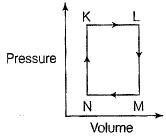Q. The succeeding operations that enable this transformation of states are (2013 Adv., Comprehension Type)

Retro (Past 13 Years) IIT - JEE Advanced (Thermodynamics) - Question 2

### A fixed mass m of a gas is subjected to transformation of states from K to L to M to N and back to K as shown in the figure.Q. The pair of isochoric processes among the transormation of states is

Retro (Past 13 Years) IIT - JEE Advanced (Thermodynamics) - Question 3

### For the process,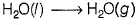at T = 100° C and 1 atm pressure, the correct choice is (2014 Adv., Single Option Correct)

Retro (Past 13 Years) IIT - JEE Advanced (Thermodynamics) - Question 4

The standard enthalpies of for mation of CO2(g), H2O (l) and glucose (s) at 25°C are - 400 kJ / mol, - 300 kJ / mol and - 1300 kJ / mol, respectively .

The standard enthalpy of combustion per gram Of glucose at 25°C is

Retro (Past 13 Years) IIT - JEE Advanced (Thermodynamics) - Question 5

Using the data provided, calculate the multiple bond energy (kJ mol-1) of a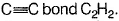That energy is (take the bond energy of a C—H bond as 350 kJ mol -1)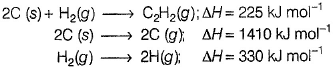(2012 , Single Option Correct)

Retro (Past 13 Years) IIT - JEE Advanced (Thermodynamics) - Question 6

Match the trans formation in Column I with appropriate options in Column II.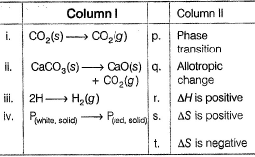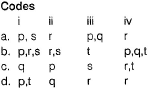*Answer can only contain numeric values
Retro (Past 13 Years) IIT - JEE Advanced (Thermodynamics) - Question 7

One mole of an ideal gas is taken from a to b along two paths denoted by the solid and the dashed lines as shown in the graph below, If the work done along the solid line path is Ws and that along the dotted line path is Wd , then the integer closest to the ratio W/ Ws is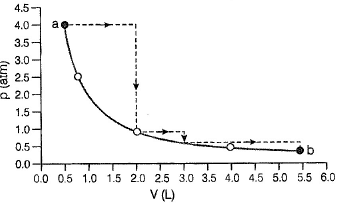Retro (Past 13 Years) IIT - JEE Advanced (Thermodynamics) - Question 8

Direction (Q. Nos. 8-9) These questions are based on Statement I and Statement II. Select the correct answer from the codes given below.

Q.

Statement I : For every chemical reaction at equilibrium , standard Gibbs ’ energy of reaction is zero.

Statement II : At constant temperature and pressure, chemical reactions are spontaneous in the direction of decreasing Gibbs ’ energy .

Retro (Past 13 Years) IIT - JEE Advanced (Thermodynamics) - Question 9

Satement I : There is a natural asymmetry between converting work to heat and converting heat to work.

Statement II : No process is possible in which the sole result is the absorption of heat from a reservoir and its complete conversion into work.

(2008, Statement Type)

Retro (Past 13 Years) IIT - JEE Advanced (Thermodynamics) - Question 10

For the process H2O (l) (1 bar, 373 K) → H2O (g) (1 bar, 373 K), the correct set of thermodynamic parameters is

(2007, Single Option Correct)

Retro (Past 13 Years) IIT - JEE Advanced (Thermodynamics) - Question 11

The value of log10 K for a reaction,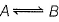is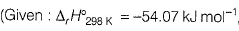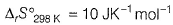and
R = 8.314 JK-1 mol-1; 2 .303 x 8.314 x 298 = 5705)

(2007, Single Option Correct)

Retro (Past 13 Years) IIT - JEE Advanced (Thermodynamics) - Question 12

The direct conversion of A to B is difficult, hence it is carried out by the following shown path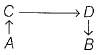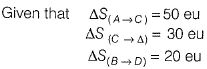where eu is entropy unit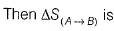(2006, Single Option Correct)

Retro (Past 13 Years) IIT - JEE Advanced (Thermodynamics) - Question 13

A monatomic ideal gas undergoes a process in which the ratio of p to V at any instant is constant and equals to 1. What is the molar heat capacity of the gas ?

Detailed Solution for Retro (Past 13 Years) IIT - JEE Advanced (Thermodynamics) - Question 13
SO3 ⇒ 3 bond pairs ⇒ sp2 hybrid ⇒ trigonal planar
BrF3 ⇒ 3 bond pairs, 2 lone pairs ⇒ sp3d hybrid ⇒ T-shaped
(SiO3)2- ⇒ 3 bond pairs ⇒ sp2 ⇒ trig. plan.
OSF2 ⇒ 3 b.p., 1 l.p. ⇒ sp3 hybrid ⇒ pyramidal.

Retro (Past 13 Years) IIT - JEE Advanced (Thermodynamics) - Question 14

One mole of monatomic ideal gas expands adiabatically at initial temperature T against a constant external pressure of 1 atm from 1 L to 2 L. Find out the final temperature (R = 0.0821 Latm K-1 mol-1)

(2005, Single Option Correct)